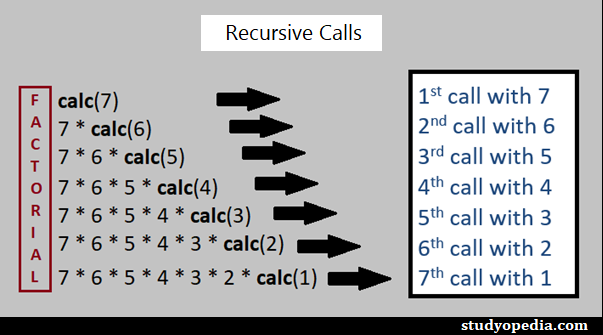## 20 Jul Java – Recursion

When a function calls itself, it is called Recursion. In another sense, with Recursion, a defined function can call itself. Recursion is a programming approach, that makes code efficient and reduces LOC.

The following figure demonstrates how recursion works when we calculate Factorial in Java with Recursion:## Recursion Example in Java

Let us now see how to find the factorial of a number in Java with Recursion:

Output

If you liked the tutorial, spread the word and share the link and our website Studyopedia with others.